# Precalculus : Find the Phase Shift of a Sine or Cosine Function

## Example Questions

### Example Question #1 : Find The Phase Shift Of A Sine Or Cosine Function

Find the phase shift of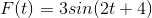.Explanation:

In the formula,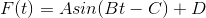.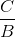represents the phase shift.

Plugging in what we know gives us: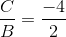.

Simplified, the phase is then.

### Example Question #1 : Find The Phase Shift Of A Sine Or Cosine Function

Describe the phase shift of the following function: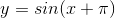Shift down byradians.

Shift up byradians.

Shift left byradians.

Vertical stretch byradians.

Shift left byradians.

Explanation:

Sinceis being added inside the parentheses, there will be a horizontal shift. The goal is to maintain zero within the parentheses so you will shift leftradians.

### Example Question #1 : Find The Phase Shift Of A Sine Or Cosine Function

Which equation would produce this graph?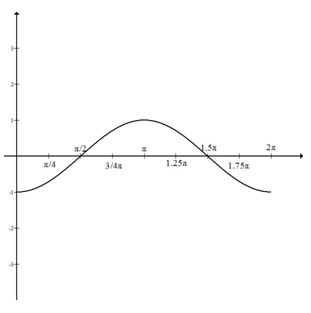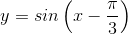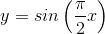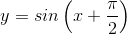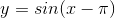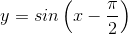Explanation:

This is the graph of sine, but shifted to the right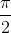units. To reflect this shift,should be subtracted from x.

Thus resulting in.

### Example Question #1 : Find The Phase Shift Of A Sine Or Cosine Function

Which equation would produce this sine graph?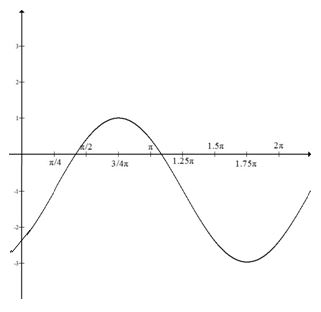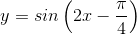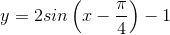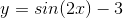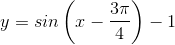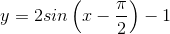Explanation:

The graph has an amplitude of 2 but has been shifted down 1: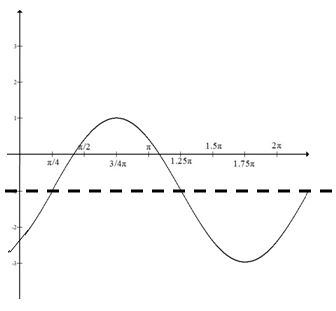In terms of the equation, this puts a 2 in front of sin, and -1 at the end.

This makes it easier to see that the graph starts [is at 0] where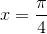.

The phase shift is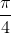to the right, or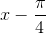### Example Question #5 : Find The Phase Shift Of A Sine Or Cosine Function

Write the equation for a sine graph with a maximum at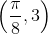and a minimum at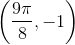.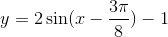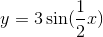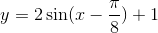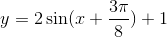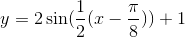Explanation:

To write this equation, it is helpful to sketch a graph: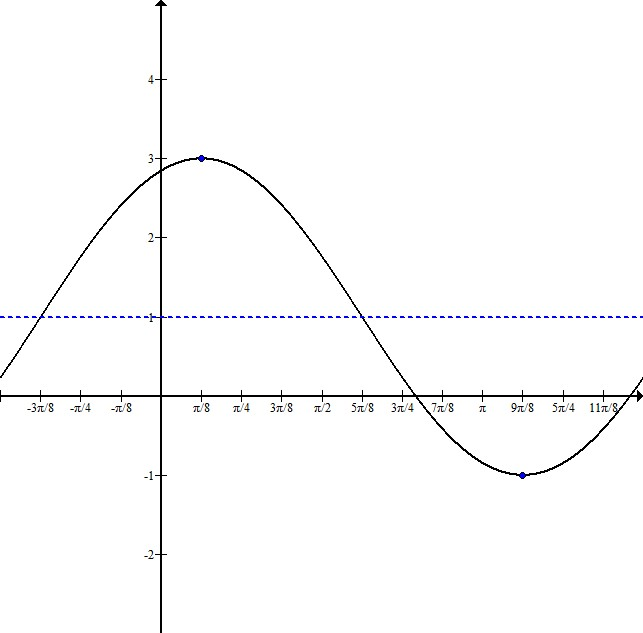Indicating the maximum and minimum points, we can see that this graph has been shifted up 1, and it has an amplitude of 2.

The distance from the maximum to the minimum point is half the wavelength. In this case, the wavelength is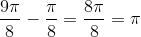. That means the full wavelength is, and the frequency is 1.

This sketch shows that the graph starts to the left of the y-axis. To figure out exactly where, subtractfrom the maximum x-coordinate,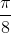: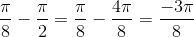.

Our equation will be in the form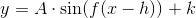where A is the amplitude, f is the frequency, h is the horizontal shift, and k is the vertical shift.

This graph has an equation of.

### Example Question #1 : Find The Phase Shift Of A Sine Or Cosine Function

Write the equation for a cosine graph with a maximum at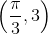and a minimum at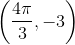.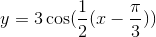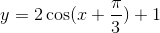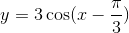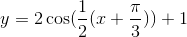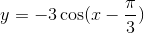Explanation:

In order to write this equation, it is helpful to sketch a graph: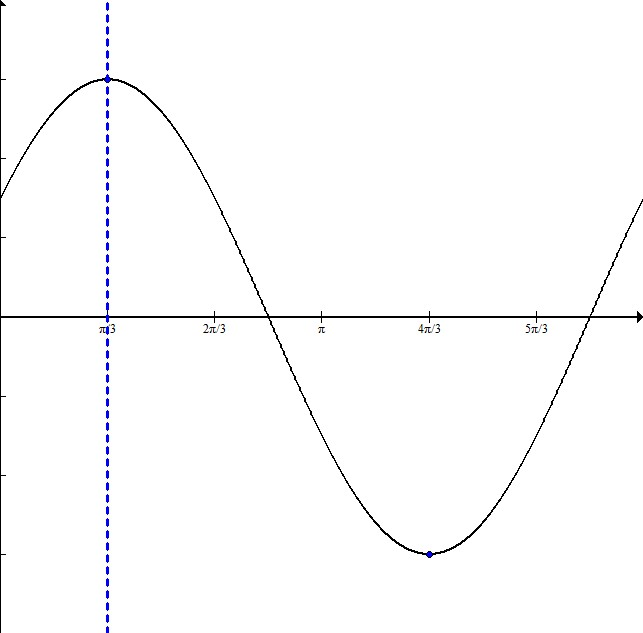The dotted line is at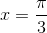, where the maximum occurs and therefore where the graph starts. This means that the graph is shifted to the right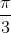The distance from the maximum to the minimum is half the entire wavelength. Here it is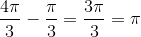.

Since half the wavelength is, that means the full wavelength isso the frequency is just 1.

The amplitude is 3 because the graph goes symmetrically from -3 to 3.

The equation will be in the form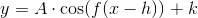where A is the amplitude, f is the frequency, h is the horizontal shift, and k is the vertical shift.

This equation is.

### Example Question #7 : Find The Phase Shift Of A Sine Or Cosine Function

Write the equation for a sine function with a maximum at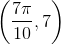and a minimum at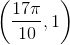.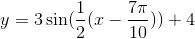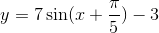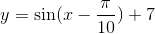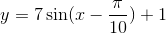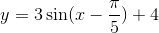Explanation:

The equation will be in the form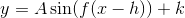where A is the amplitude, f is the frequency, h is the horizontal shift, and k is the vertical shift.

To write the equation, it is helpful to sketch a graph: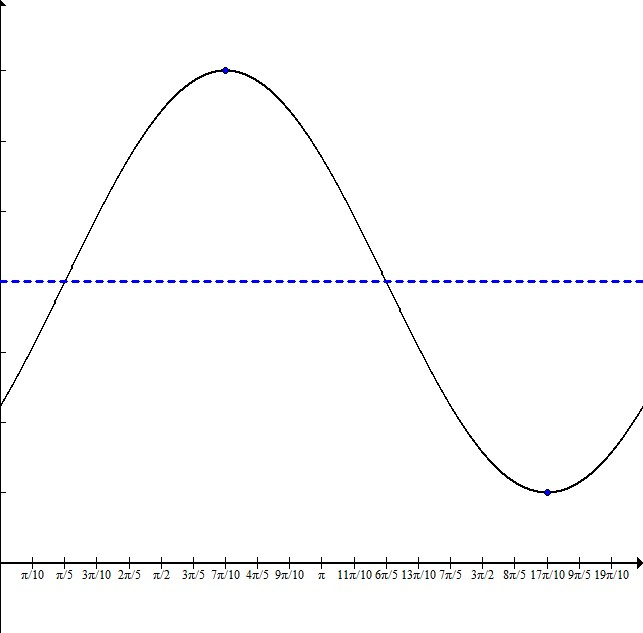From plotting the maximum and minimum, we can see that the graph is centered on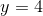with an amplitude of 3.

The distance from the maximum to the minimum is half the wavelength. For this graph, this distance is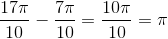.

This means that the total wavelength isand the frequency is 1.

The graph startsbehind the maximum point. To determine this x value, subtractfrom the x-coordinate of the maximum: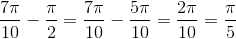Our equation is:.

### All Precalculus Resources